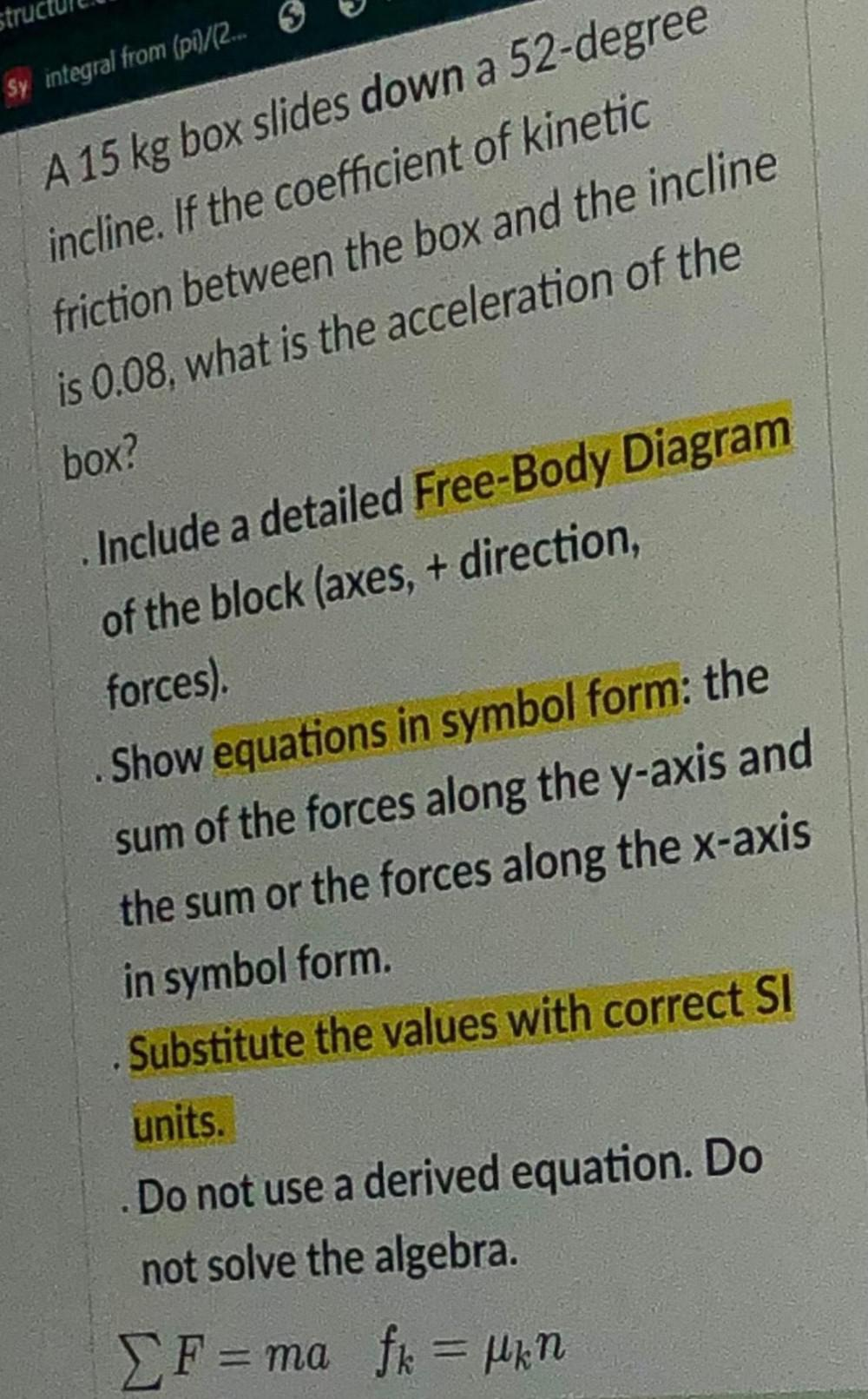Question:

# struc Sy integral from (pi)/(2. 6 A 15 kg box slides down a 52-degree incline. If the coefficient of kinetic friction between thstruc Sy integral from (pi)/(2. 6 A 15 kg box slides down a 52-degree incline. If the coefficient of kinetic friction between the box and the incline is 0.08, what is the acceleration of the box? Include a detailed Free-Body Diagram of the block (axes,+ direction, forces). Show equations in symbol form: the sum of the forces along the y-axis and the sum or the forces along the x-axis in symbol form. Substitute the values with correct SI units. Do not use a derived equation. Do not solve the algebra. F=ma fk = ukin